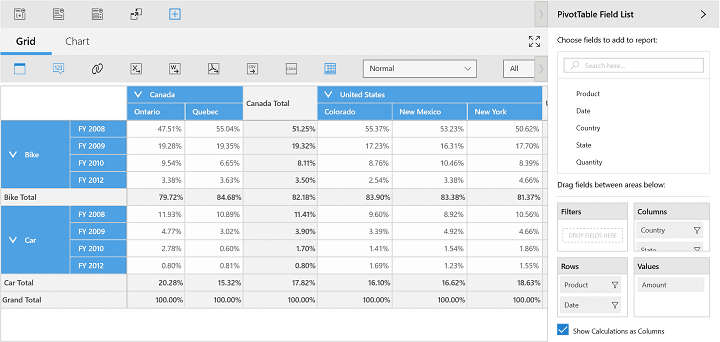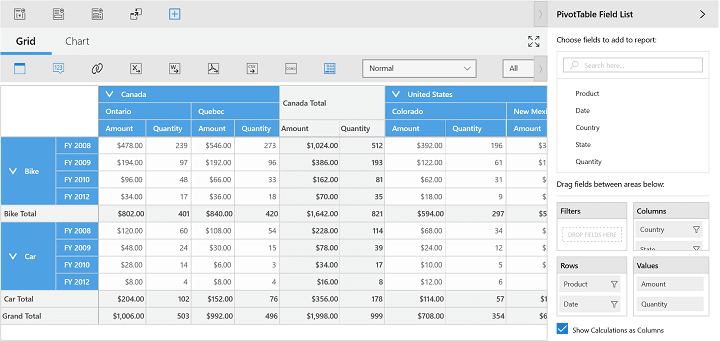# Custom Calculations in UWP Pivot Client (SfPivotClient)

10 May 20214 minutes to read

CalculationType is an enumerator, used to specify the type of the calculation; it is defined in the PivotComputationInfo class. Various types of calculations are used to perform calculations as specified below:

• NoCalculation: Removes the custom calculations and restores the original values (default values). Pivot values are displayed as default values.
• PercentageOfGrandTotal: Displays a value cell as a percentage of grand total for all value cells of the pivot engine.
• PercentageOfColumnTotal: Displays all value cells in each column as a percentage of its corresponding column total.
• PercentageOfRowTotal: Displays all value cells in each row as a percentage of its corresponding row total.
• PercentageOfParentColumnTotal: Displays a value cell as a percentage of parent column item values.
• PercentageOfParentRowTotal: Displays a value cell as a percentage of parent row item values.
• PercentageOfParentTotal: Displays a value cell as a percentage of the base field (parent row or column total).
• Index: Displays a value cell as an index value based on the pivot engine generation.
• Formula: Displays a calculation based on a well-formed algebraic expression involving other calculations.
• PercentageOf: Displays a value as a percentage of base item value in the base field.
• DifferenceFrom: Displays a value as the difference from the value of the base item in the base field.
• PercentageOfDifferenceFrom: Displays value as the percentage difference from the value of base item in the base field.
• RunningTotalIn: Displays the value for successive items in the base field as a running total.
• PercentageOfRunningTotalIn: Calculates the value for successive items in the base field that are displayed as a running total in a percentage.
• RankSmallestToLargest: Displays rank of selected values in a specific field and lists the smallest item in the field as 1. Larger values will have higher rank values.
• RankLargestToSmallest: Displays ranks of selected values in a specific field and lists the largest item in the field as 1. Smaller values will have higher rank values.
• Distinct: Displays the subtotals based on distinct values of the base item that is defined for the calculation item.

The following code snippet illustrates how to specify the calculation type for pivot calculations.

``````<syncfusion:SfPivotClient Name="PivotClient1" ItemSource="{Binding ProductSalesData}"
PivotRows="{Binding PivotRows}" PivotColumns="{Binding PivotColumns}">
<syncfusion:SfPivotClient.PivotCalculations>
<syncfusion:PivotComputationInfo CalculationName="Total" FieldName="Amount" Format="C" CalculationType="PercentageOfColumnTotal"/>
</syncfusion:SfPivotClient.PivotCalculations>
</syncfusion:SfPivotClient>``````
``````PivotClient1.PivotCalculations.Add(new PivotComputationInfo
{
FieldName = "Amount",
Format = "C",
CalculationType = CalculationType.PercentageOfColumnTotal
});``````
``````PivotClient1.PivotCalculations.Add(New PivotComputationInfo() With
{ _
Key .FieldName = "Amount", _
Key .Format = "C", _
Key .CalculationType = CalculationType.PercentageOfColumnTotal _
})``````## Providing expression field calculation for summaries

The SfPivotClient supports the calculated field for summary cells despite any options provided in summaries such as sum, count, maximum, minimum, etc. This support can be achieved by setting the calculation type as formula and specifying the appropriate formula as specified in the following code snippet.

``````<syncfusion:SfPivotClient Name="PivotClient1" ItemSource="{Binding ProductSalesData}"
PivotRows="{Binding PivotRows}" PivotColumns="{Binding PivotColumns}">
<syncfusion:SfPivotClient.PivotCalculations>
<syncfusion:PivotComputationInfo CalculationName="Total" FieldName="Amount" Format="C" CalculationType="Formula" Formula="Quantity * 2"/>
<syncfusion:PivotComputationInfo CalculationName="Total" FieldName="Quantity" Format"#.##" />
</syncfusion:SfPivotClient.PivotCalculations>
</syncfusion:SfPivotClient>``````
``````PivotClient1.PivotCalculations.Add(new PivotComputationInfo { FieldName = "Amount", Format = "C", CalculationType = CalculationType.Formula, Formula = "Quantity * 2" });
PivotClient1.PivotCalculations.Add(new PivotComputationInfo { FieldName = "Quantity", Format = "#.##" });``````
``````PivotClient1.PivotCalculations.Add(New PivotComputationInfo() With { _
Key .FieldName = "Amount", _
Key .Format = "C", _
Key .CalculationType = CalculationType.Formula, _
Key .Formula = "Quantity * 2" _
})
PivotClient1.PivotCalculations.Add(New PivotComputationInfo() With { _
Key .FieldName = "Quantity", _
Key .Format = "#.##" _
})``````